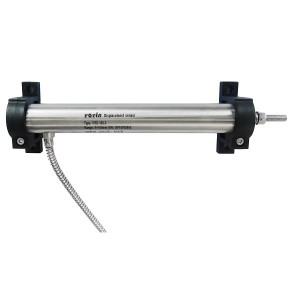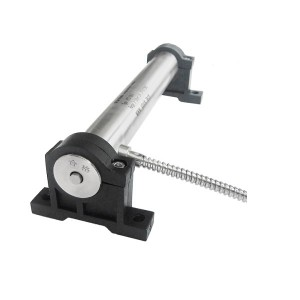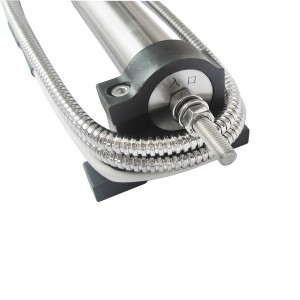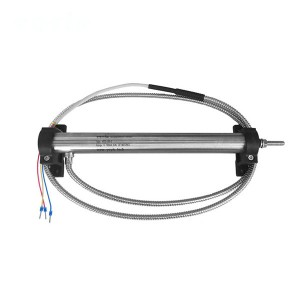# How to Calculate the Sensitivity of Displacement Sensor HTD-50-6?

To calculate the sensitivity of a linear displacement sensor HTD-50-6, you usually need to know the following two parameters:• The variation of the output signal of the sensor (e.g. voltage or current).
• Corresponding displacement variation (e.g. length or position).The sensor sensitivity can be determined by calculating the ratio between the output signal variation and the displacement variation. The specific calculation method is as follows: sensitivity=output signal variation/displacement variationFor example, if the output signal of a linear position sensor HTD-50-6 changes by 10 mV when the displacement changes by 1 mm, the sensitivity can be calculated as: Sensitivity=10 mV/1 mm=10 mV/mm.

The unit of sensitivity will depend on the unit of output signal and displacement, for example, in millivolts per millimeter in the above example.It should be noted that for some LVDT displacement sensor HTD-50-6, the sensitivity may not be constant, but varies with the displacement range. In this case, the local sensitivity can be calculated using the output signal variation and displacement variation at different positions or displacement points, or the overall sensitivity can be estimated using the average value.

• Previous:
• Next:

• Post time: Sep-15-2023